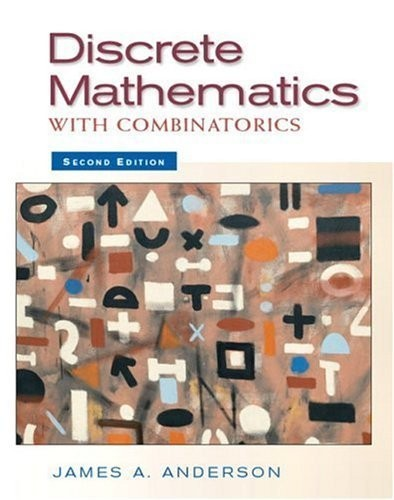# Discrete Mathematics with Combinatorics by James A Anderson (University of South Carolina Spartanburg)

In Stock
£33.99
Placing each topic in context, this text covers concepts associated with discrete mathematical systems that have applications in computer science, engineering, and mathematics. It introduces basic concepts and also covers graphs, trees, recursion, algebra, theory of computing, and combinatorics. It contains many examples to reinforce the concepts.
Only 1 left

## Discrete Mathematics with Combinatorics Summary

### Discrete Mathematics with Combinatorics by James A Anderson (University of South Carolina Spartanburg)

For one-/two- semester, freshman courses in Discrete Mathematics.

This carefully organized, very readable text covers every essential topic in discrete mathematics in a logical fashion. Placing each topic in context, it covers concepts associated with discrete mathematical systems that have applications in computer science, engineering, and mathematics. The author introduces more basic concepts at the freshman level than are found in other texts, in a simple, accessible form. Introductory material is balanced with extensive coverage of graphs, trees, recursion, algebra, theory of computing, and combinatorics. Extensive examples throughout the text reinforce concepts.

### Why buy from World of BooksOur excellent value books literally don't cost the earthFree delivery in the UKEvery used book bought is one saved from landfill

1. Truth Tables, Logic, and Proofs.

Statements and Connectives. Conditional Statements. Equivalent Statements. Axiomatic Systems: Arguments and Proofs. Completeness in Propositional Logic. Karnaugh Maps. Circuit Diagrams.

2. Set Theory.

Introduction to Sets. Set Operations. Venn Diagrams. Boolean Algebras. Relations. Partially Ordered Sets. Equivalence Relations. Functions.

3. Logic, Integers, and Proofs.

Predicate Calculus. Basic Concepts of Proofs and the Structure of Integers. Mathematical Induction. Divisibility. Prime Integers. Congruence Relations.

4. Functions and Matrices.

Functions. Special Functions. Matrices. Cardinality. Cardinals Revisited.

5. Algorithms and Recursion.

The "for" Procedure and Algorithms for Matrices. Recursive Functions and Algorithms. Complexity of Algorithms. Sorting Algorithms. Prefix and Suffix Notation. Binary and Hexadecimal Numbers. Signed Numbers. Matrices Continued.

6. Graphs, Directed Graphs and Trees.

Graphs. Directed Graphs. Trees. Instant Insanity. Euler Paths and Cycles. Incidence and Adjacency Matrices. Hypercubes and Gray Code.

7. Number Theory.

Sieve of Eratosthenes. Fermat's Factorization Method. The Division and Euclidean Algorithms. Continued Fractions. Convergents.

8. Counting and Probability.

Basic Counting Principles. Inclusion-Exclusion Introduced. Permutations and Combinations. Generating Permutations and Combinations. Probability Introduced. Generalized Permutations and Combinations. Permutations and Combinations with Repetition. Pigeonhole Principle. Probability Revisited. Bayes' Theorem. Markov Chains.

9. Algebraic Structures.

Partially Ordered Sets Revisited. Semigroups and Semilattices. Lattices. Groups. Groups and Homomorphisms. Linear Algebra.

10. Number Theory Revisited.

Integral Solutions of Linear Equations. Solutions of Congruence Equations. Chinese Remainder Theorem. Order of an Integer.

11. Recursion Revisited.

Homogeneous Linear Recurrence Relations. Nonhomogeneous Linear Recurrence Relations. Finite Differences. Factorial Polynomials. Sums of Differences.

12. Counting Continued.

Occupancy Problems. Catalan Numbers. General Inclusion-Exclusion and Derangements. Rook Polynomials and Forbidden Positions.

13. Generating Functions.

Defining the Generating Function (optional). Generating Functions and Recurrence Relations. Generating Functions and Counting. Partitions. Exponential Generating Functions.

14. Graphs Revisited.

Algebraic Properties of Graphs. Planar Graphs. Coloring Graphs. Hamiltonian Paths and Cycles. Weighted Graphs and Shortest Path Algorithms.

15. Trees.

Properties of Trees. Binary Search Trees. Weighted Trees. Traversing Binary Trees. Spanning Trees. Minimal Spanning Trees.

16. Networks.

Networks and Flows. Matching. Petri Nets.

17. Theory of Computation.

Regular Languages. Automata. Finite State Machines with Output. Grammars. Turing Machines.

18. Theory of Codes.

Introduction. Generator Matrices. Hamming Codes.

19. Enumeration of Colors.

Burnside's Theorem. Polya's Theorem.

20. Rings, Integral Domains, and Fields.

Rings and Integral Domains. Integral Domains. Polynomials. Algebra and Polynomials.

21. Group and Semigroup Characters.

Complex Numbers. Group Characters. Semigroup Characters.

22. Applications of Number Theory.

Application: Pattern Matching. Application: Hashing Functions. Application: Cryptography.

Bibliography.

Hints and Solutions to Selected Exercises.

Index.

GOR002789519
Discrete Mathematics with Combinatorics by James A Anderson (University of South Carolina Spartanburg)
James A Anderson (University of South Carolina Spartanburg)
Used - Good
Paperback
Pearson Education (US)
2003-08-19
928
0130457914
9780130457912
N/A
Book picture is for illustrative purposes only, actual binding, cover or edition may vary.
The book has been read but remains in clean condition. All pages are intact and the cover is intact. Some minor wear to the spine.